Physic Tutorials

# Notes on alternating Current through a Capacitor and Inductor

Notes on alternating Current through a Capacitor and Inductor

A.C through a capacitorAn alternating voltage V = Vo sin wt when applied across a capacitor C, the current is given by: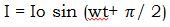Both the experiment and theory show that the voltage (V) and the current (I) are out of phase. The current leads the voltage by 90 degree i.e. the current is ahead by the voltage. The voltage is said to lag on the current.

Capacitance Reactance Xc

The opposition offered by the capacitor to the flow of alternating current is known as the capacitance reactance Xc.Peak and r.m.s values of A.C

The amplitude or peak value of the current Io is the maximum value of the current. The root mean square is that steady current that will develop the same quantity of heat, at the same time, and at the same resistance.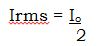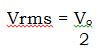Inductance in Alternating Current Circuit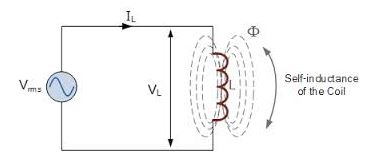When an inductor is connected to an alternating voltage source, VL = Vo sin wt and the current

I is given by IL = Iosin (wt)

The current is delayed behind the voltage in the circuit i.e the current lags the voltage by 90 degree.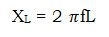Where the unit of L is Henry (H), f is in Hertz and XL in ohms

Resistance (R) and, Inductor (L) series circuit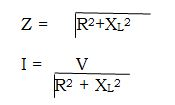Capacitor- Resistor (C-R) series circuitSeries Circuit containing Resistance (R), Inductance (L) and Capacitance C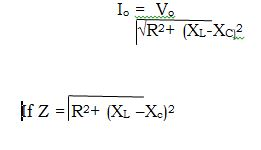Example1

In an A.C circuit, if the peak voltage and current are 330volts and 80A what is the value of the root mean square voltage and current

SolutionExample 2

A series circuit consists of a resistance 600 ohms and an inductance of 5 Henry’s An A.C voltage of 15 volts (r.m.s) and frequency 50Hz is applied across the series circuit calculate

1. The current flowing through the circuit
2. The voltage across the inductor

solution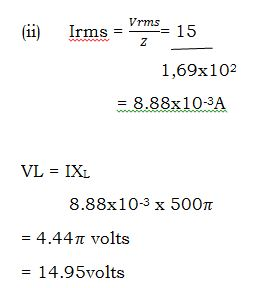Recommended: Solved questions on alternating current

### Bolarinwa Olajire

A lecturer, Educationist, PhD student at FUNAAB, and a Blogger.

### One Comment

1.Timothy says:

I love this website